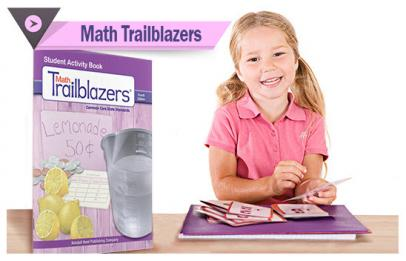# Product Spotlight: Math Trailblazers

### December 22, 2016By: Kendall Hunt

Math Trailblazers® 4th Edition is a coherent, research-based, K–5 mathematics curriculum that reflects the goals and ideas of the National Council of Teachers of Mathematics (NCTM) Principles and Standards for School Mathematics and aligns with the Common Core State Standards.  Math Trailblazers is an elegantly engineered, inquiry-based curriculum that makes mathematics engaging and meaningful to students. Math Trailblazers is based on more than 25 years of National Science Foundation funded research by experts at the Teaching Integrated Mathematics and Science (TIMS) Project and the Learning Sciences Research Institute of the University of Illinois at Chicago. Math Trailblazers remains a comprehensive and rigorous curriculum rich in hands-on activities, lively discussion, and cooperative opportunities for learning.  The 4th edition in particular is designed to focus on teacher as learner so that all classrooms are mathematically confident.

Program Features

Kindergarten:

• Activities, discussions, and explorations to develop understanding with concepts related to whole number and sets of objects (representing, relating, and operating on whole numbers).

• Experiences to develop ideas surrounding descriptions of shapes and space.

Math Trailblazers is built upon the tenet that children develop knowledge of math concepts through their interactions with people and things.  Mathematical concepts are developed through play, the solving of realistic problems, open-ended activities and a variety of story situations and representations. It provides all the resources teachers need to prepare their kindergarten classroom, meet the Common Core State Standards, and create a formal learning environment where students can apply prior mathematical knowledge to discover, explore, and develop concepts.

• Students develop strategies for adding and subtracting whole numbers. They use direct models, counting strategies, and reasoning strategies to model and solve a variety of join, separate, part-whole, and compare problems.

• Using these strategies, students further develop their understanding of the properties of and relationship between addition and subtraction.

• Students develop an understanding of the unit of 10 as they compose and decompose numbers through 200.

• They develop strategies to add and subtract multiples of ten. Students then compare and problem-solve with these larger numbers.

• Students use this understanding to measure length.

•  Students analyze and describe two-dimensional and three-dimensional shapes, and partition shapes into equal shares to solve problems.

• Students continuing to develop reasoning strategies allowing them to become more fluent with addition and subtraction facts.

• Students extend their understanding of the unit of 10 and of the base-ten number system to develop place-value concepts.

• Using place-value concepts and the properties of addition and subtraction to develop mental math strategies, conceptual models, and standard algorithms for multi-digit addition and subtraction.

• Students develop methods for measuring and comparing lengths measured in different-sized standard and nonstandard units.

• Students using equal groups of objects or equal partitions of a shape to develop foundations for multiplication and division.

• Students applying place value concepts to continue developing fluency and competency with multi-step, multi-digit addition and subtraction problems.

• Students use of mental math strategies, conceptual models, and paper-and-pencil procedures to solve multiplication and division problems.

• Making connections using drawings, number lines, rectangular arrays, tables, and graphs to reason about multiplication and division and the relationship between them.

• Developing efficient strategies for the multiplication facts.

• Students developing models for comparing and finding equivalent fractions.

• Describing, analyzing, and classifying polygons and polyhedra using their properties.

• Students developing mental math strategies, conceptual models, and standard algorithms for multi-digit multiplication and division. The rectangular array model is shown along with how to use graphs and tables to reason with multiplication and division.

• Deepening student's understanding of and flexibility with large numbers and addition and subtraction operations.

•  Developing models for comparing, finding equivalence, and adding, subtracting, and multiplying fractions by using and connecting multiple representations.

• Students describing, analyzing, and classifying lines, angles, and polygons using their properties.

• Students using multiple representations and real-world contexts to continue to support their development of fraction concepts and operations.

• The use of graphs, tables, and stories to interpret ratios—a fraction as a multiplicative relationship between two numbers.

• Students using these relationships to make generalizations, problem solve, and compare units of measure.

• Students continuing to develop and apply a variety of strategies for solving multi-step problems involving addition, subtraction, multiplication, and division.

• Extending whole number and fractional representations, strategies, and procedures to models of decimals.

• Classifying two-dimensional shapes using properties.